##### Organic Chemistry I Workbook For Dummies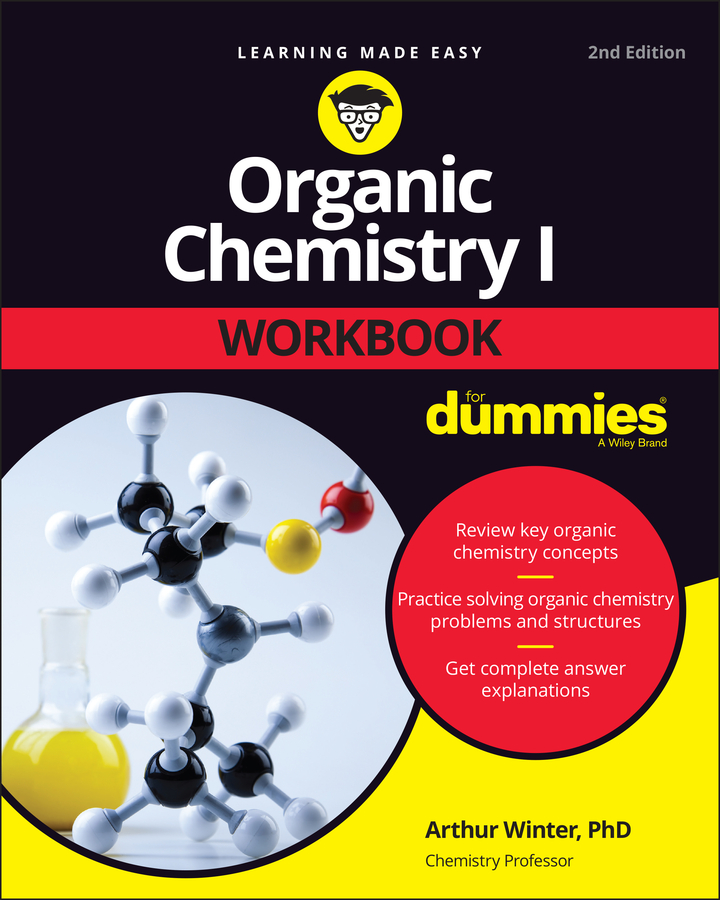Real reagents (reactants) tend to be measured in units of mass or volume. Real products are measured in the same way. So you need to be able to use mole-mass, mole-volume, and mole-particle conversion factors to translate between these different dialects of counting.

The figure summarizes the interrelationship among all these things and serves as a flowchart for problem-solving. All roads lead to and from the mole.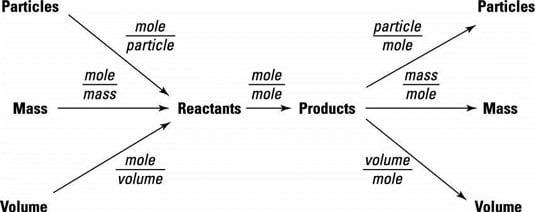A problem-solving flowchart showing the use of mole-mole, mole-mass, mole-volume, and mole-particle conversion factors.

If you look at the figure, you can see that it isn't possible to convert directly between the mass of one substance and the mass of another substance. You must convert to moles and then use the mole-mole conversion factor before converting to the mass of a new substance. The same can be said for conversions from the particles or volume of one substance to that of another substance. The mole is always the intermediary you use for the conversion.

Do all your calculations in one long stoichiometric problem, saving any rounding for the end. When you have a string of conversion factors, you're far better off calculating everything in one step instead of breaking up a problem into multiple steps where you type a calculation into your calculator, hit enter, and then move on to the next step. Doing that leads to a greater degree of error.

Try an example: Calcium carbonate decomposes to produce solid calcium oxide and carbon dioxide gas according to the following reaction.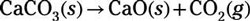Assuming that 10.0 g of calcium carbonate decomposes, answer the two following questions:

1. How many grams of calcium oxide are produced?

First, convert 10.0 g of calcium carbonate to moles of calcium carbonate by using the molar mass of calcium carbonate (100.09 g/mol) as a conversion factor. To determine the grams of calcium oxide produced, you must then convert from moles of calcium carbonate to moles of calcium oxide. Keep in mind that you take the number of moles for the mole-mole conversion from the coefficients in the balanced chemical equation. Then convert from moles of calcium oxide to grams of calcium oxide by using the molar mass of calcium oxide (56.08 g/mol) as a conversion factor: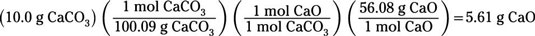You find that 5.61 g of CaO are produced.

2. At standard temperature and pressure (STP), how many liters of carbon dioxide are produced?

To determine the liters of carbon dioxide produced, follow the initial mass-mole conversion with a mole-mole conversion to find the moles of carbon dioxide produced. Then convert from moles of carbon dioxide to liters by using the fact that at STP, each mole of gas occupies 22.4 L: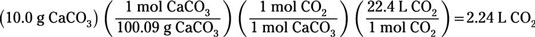You calculate that 2.24 L of CO2 are produced.

Notice how both calculations require you to first convert to moles and then perform a mole-mole conversion using stoichiometry from the reaction equation. Then you convert to the desired units. Both solutions consist of a chain of conversion factors, each factor bringing the units one step closer to those needed in the answer.